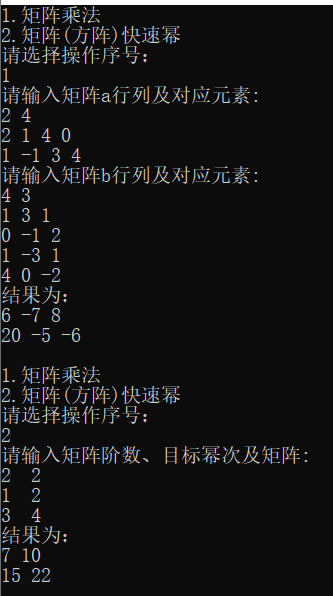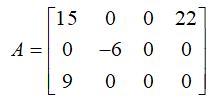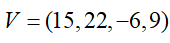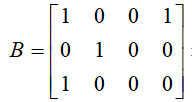• matrix Matrix_Multiply(matrix a, matrix b, int n, int m, int t) //实现矩阵乘法，输入两个矩阵必须合法， 前者 n 行 m 列，后者 m 行 t 列 { matrix c; int i, j, k; for(i = 0; i ; ++i) c.x[i] = c.x[i...
本来是为了偷个懒，，，可是
计算能力一定要好好练！
#include <iostream>
#include <cstdio>
#include <algorithm>
#include <cstring>
#define mod 1000000007
using namespace std;
const int N = 36;

struct matrix
{
int x[N][N];
};

matrix Matrix_Multiply(matrix a, matrix b, int n, int m, int t) //实现矩阵乘法，输入两个矩阵必须合法， 前者 n 行 m 列，后者 m 行 t 列
{
matrix c;
int i, j, k;
for(i = 0; i <= N; ++i)
c.x[i] = c.x[i] = 0;
for(i = 1; i <= n; ++i)
for(j = 1; j <= t; ++j)
{
int sum = 0;
for(k = 1; k <= m; ++k)
sum += a.x[i][k]*b.x[k][j]%mod;
c.x[i][j] = sum;
}
return c;
}

matrix mul2(matrix a, matrix b, int n) //两个 n 阶矩阵相乘时， 也可以用这个
{
matrix c;
int i, j, k;
for(i = 0; i <= n; ++i)
c.x[i] = c.x[i] = 0;
for(i = 1; i <= n; ++i)
for(j = 1; j <= n; ++j)
{
int sum = 0;
for(k = 1; k <= n; ++k)
sum += a.x[i][k]*b.x[k][j];
c.x[i][j] = sum;
}
return c;
}

matrix Matrix_fastpower(matrix a, int n, int m) //矩阵 a , 阶数 n , 指数 m
{
matrix c;
int i, j, k;
for(i = 0; i <= n; ++i)
for(j = 0; j <= n; ++j)
{
c.x[i][j] = 0;
if(i == j)
c.x[i][j] = 1;
}
if(m == 0)
return c;
if(m == 1)
return a;
while(m)
{
if(m&1)
c = mul2(a, c, n);
a = mul2(a, a, n);
m >>= 1;
}
return c;
}

matrix a, b, ans;

int main()
{
int n, m, t, i, j, k, num;
printf("1.矩阵乘法\n2.矩阵(方阵)快速幂\n请选择操作序号：\n");
while(~scanf("%d", &num))
{
memset(a.x, 0, sizeof a.x);
memset(b.x, 0, sizeof b.x);
memset(ans.x, 0, sizeof ans.x);
if(num == 1)
{
printf("请输入矩阵a行列及对应元素:\n");
scanf("%d%d", &n, &m);
for(i = 1; i <= n; ++i)
for(j = 1; j <= m; ++j)
scanf("%d", &a.x[i][j]);
printf("请输入矩阵b行列及对应元素:\n");
scanf("%d%d", &m, &t);
for(i = 1; i <= m; ++i)
for(j = 1; j <= t; ++j)
scanf("%d", &b.x[i][j]);
ans = Matrix_Multiply(a, b, n, m, t);
printf("结果为：\n");
for(i = 1; i <= n; ++i)
{
int flag = 0;
for(j = 1; j <= t; ++j)
{
if(flag)
printf(" %d", ans.x[i][j]);
else
printf("%d", ans.x[i][j]);
flag = 1;
}
printf("\n");
}
}
if(num == 2)
{
printf("请输入矩阵阶数、目标幂次及矩阵:\n");
scanf("%d%d", &n, &m);
for(i = 1; i <= n; ++i)
for(j = 1; j <= n; ++j)
scanf("%d", &a.x[i][j]);
if(n != 1)
{
ans = Matrix_fastpower(a, n, m);
printf("结果为：\n");
for(i = 1; i <= n; ++i)
{
int flag = 0;
for(j = 1; j <= n; ++j)
{
if(flag)
printf(" %d", ans.x[i][j]);
else
printf("%d", ans.x[i][j]);
flag = 1;
}
printf("\n");
}
}
else
{
int tp = a.x;
int anss = 1;
for(i = 1; i <= m; ++i)
anss *= tp;
printf("结果为：\n");
printf("%d\n", anss);
}
}
printf("\n1.矩阵乘法\n2.矩阵(方阵)快速幂\n请选择操作序号：\n");
}
return 0;
}


运行结果（使用方法）展开全文• ## c语言实现矩阵运算

千次阅读 2020-06-12 20:08:49
所以我决定写一写自己的编程过程，希望能有些帮助。 1.一个矩阵要有的信息包括它的行数列数以及每一个元素的信息，所以我们设置了一个结构体来包含这些信息，结构体的成员就是整型的行数和列数和一个表示元素内容的...
前言：我知道一定会有郫县男子职业技术学院的同学看到这个，之前我一直在想要不要直接把程序放在这，因为有些课程确实操蛋，但后来想了想，或许这就是痛苦学习法吧。所以我决定写一写自己的编程过程，希望能有些帮助。
1.一个矩阵要有的信息包括它的行数列数以及每一个元素的信息，所以我们设置了一个结构体来包含这些信息，结构体的成员就是整型的行数和列数和一个表示元素内容的二级指针。其中二级指针比较难以理解，二级指针是指向一级指针的，一级指针指向具体的值，这里我们可以认为这个值就是具体的元素内容。我同学璐璐给我打了个比喻：甲是一个二级指针，他知道一个房间号A，这个房间A里的人乙是一个一级指针，而乙又知道一个房间号B。假设房间号A，B是变量，那么甲按照他知道的A就会进入不同的房间找到不同的乙，这个过程就相当于矩阵寻行，即找到第几行，我们要做的就是修改A的值，即甲知道的房间号，这样就可以改变找到的行数，体现在程序里就是改变二级指针指向的内容（改变二级指针的地址）。然后在甲知道的房间号A不变的情况下，改变乙知道的房间号B，就是在某一行内寻列，即找到某一列，至此得到的房间B里的人就是你要的元素。
2.解决了二级指针的问题，接下来就是结构体分配内存的问题了，我也不会，所以我要去查。。。。
A few hours later…
（1）首先我们要明白，定义一个结构体就相当于定义了一个新的变量类型，当你使用完typedef之后，你可以像使用int一样使用你定义的结构体。如上图我定义了一个结构体Matrix,那么我可以使用Matrix t;来定义一个结构体变量t。
后来我发现在子函数里还需要为矩阵分配内存，就不太明白为什么在Matrix t;之后还要分配内存（定义变量过程中会根据变量类型分配内存）问了亲爱的璐璐之后就明白了：
定义变量过程中为二级指针和两个整形变量分配了内存，但是我使用矩阵还需要内存用来存储一级指针的值和元素值，也就是我还需要安排前述1中的房间A和房间B。
（2）我们来看看下边这个子函数
void InitialMatrix(Matrix* T, int row, int col) {
int i;
T->mat = (double**)malloc(row * sizeof(double*));
for (i = 0; i < row; i++)
T->mat[i] = (double*)malloc(col * sizeof(double));
T->row = row;
T->col = col;
}

从主函数传递矩阵是通过矩阵的首地址来完成的
 Matrix t1;
InitialMatrix(&t1, r, c);

我们将主函数定义的结构体的首地址传到子函数中就相当于将矩阵传到了子函数，子函数输入形参中的结构体指针T就指向传入的结构体，我之前一直以为是将结构体的首地址存储在了指针T所指向的地址中，后来才明白是直接将结构体的首地址赋给了指针T，所以此时T代表的就是结构体的首地址，而*T代表的是一个符号，与主函数中的t1是一样的。
在子函数中：
 T->mat = (double**)malloc(row * sizeof(double*));

上述过程就是在为结构体中的二级指针赋值，赋的值是分配内存的一级指针的首地址。注意这里T->mat表示结构体指针对应的结构体的成员mat，根据前述结构体定义可知成员mat是一个二级指针，对他赋值为一级指针的首地址是合理的,然后要明白上述语句其实就相当于创建了一个数组，T->mat就是数组的首地址。
最后还有一个我一直不清楚的问题，被曹巨一语道破：
*T.mat和T->mat是一样的
‘.’是结构体变量用来找子元素的
‘->’是结构体指针用来找子元素的
这里的结构体指针和结构体变量就是之前说的[ T ]和[ *T ]的区别
3.在后边编辑矩阵释放内存子程序的时候，我又遇到了一个问题，就是在定义矩阵的时候分配内存分了两步，先为一级指针分配内存，再为矩阵元素分配内存，所以我就在想可不可以直接对矩阵名进行释放，后来我去查了查觉得应该不行，这里牵扯到堆和栈（我也没学过）但是也能理解
大家可以自己去看看：
https://blog.csdn.net/r91987/article/details/6337032


展开全文指针
• 编程实现上述表示法的矩阵加法运算。 输入格式 首先输入两个整数n和m，为矩阵维数，然后输入两个矩阵的值B1、V1和B2、V2。 输出格式 输出矩阵运算结果B、V。 样例输入 3 4 1 0 0 1 0 1 0 0 1 0 0 0 15 22 ...
问题描述

将稀疏矩阵A的非零元以行序为主序的顺序存于一维数组V中，并用二维数组B表示A中的相应元素是否为零元素，例如：可用和表示。

编程实现上述表示法的矩阵加法运算。

输入格式

首先输入两个整数n和m，为矩阵维数，然后输入两个矩阵的值B1、V1和B2、V2。

输出格式

输出矩阵运算结果B、V。

样例输入

3 4

1 0 0 1

0 1 0 0

1 0 0 0

15 22 -6 9

0 1 1 0

0 1 1 0

1 0 0 1

3 2 6 2 -5 8

样例输出

1 1 1 1

0 0 1 0

1 0 0 1

15 3 2 22 2 4 8

#include<stdio.h>
#include<stdlib.h>
#define MAXSIZE 100
#define MAXLENGTH 10000
int main(){
int i,j;
int n,m;
int p=0, q=0, r=0;	//做加法的下标
int count1 = 0;		//记录非零元个数
int count2 = 0;
int tmp;
int B1[MAXSIZE][MAXSIZE];
int B2[MAXSIZE][MAXSIZE];
int B[MAXSIZE][MAXSIZE] = {0};
int V1[MAXLENGTH];
int V2[MAXLENGTH];
int V[MAXLENGTH] = {0};
scanf("%d %d", &n, &m);
//输入矩阵
for(i=0; i<n; i++){
for(j=0; j<m; j++){
scanf("%d", &tmp);
if(tmp == 1)
count1 ++;
B1[i][j] = tmp;
}
}
for(i=0; i<count1; i++){
scanf("%d", &V1[i]);
}
for(i=0; i<n; i++){
for(j=0; j<m; j++){
scanf("%d", &tmp);
if(tmp == 1)
count2 ++;
B2[i][j] = tmp;
}
}
for(i=0; i<count2; i++){
scanf("%d", &V2[i]);
}
//做加法
for(i=0; i<n; i++){
for(j=0; j<m; j++){
if(B1[i][j] == 1 && B2[i][j] == 0){
B[i][j] = 1;
V[p] = V1[q];
p++;
q++;
}else if(B1[i][j] == 0 && B2[i][j] == 1){
B[i][j] = 1;
V[p] = V2[r];
p++;
r++;
}else if(B1[i][j] == 1 && B2[i][j] == 1){
tmp = V1[q] + V2[r];
q++; r++;
if(tmp != 0){
B[i][j] = 1;
V[p] = tmp;
p++;

}
}
}
}
//输出
for(i=0; i<n; i++){
for(j=0; j<m; j++){
printf("%d ", B[i][j]);
}
printf("\n");
}
i=0;
while(1){
if(V[i] == 0)
break;
printf("%d ", V[i]);
i++;
}
return 0;
} 


展开全文数据结构
• 本文介绍了用C语言编程及相关定理实现对非周期马尔可夫链遍历性及其遍历步数的判断和计算方法,并阐明了其理论根据。它的最大特点是避免了矩阵乘法的繁复运算,计算快速准确。
• 基本信息 · 开本： 16 · 出版日期： 2004-01 ...实例95　人工神经网络的C语言实现 实例96　K_均值算法 实例97　ISODATA算法 实例98　快速傅立叶变换 实例99　求解野人与传教士问题 实例100　简单专家系统
• 大电网的等值电路参数计算、导纳矩阵存储、潮流计算等都需要在电脑上编程实现。 打算试试用拿手的纯C来实现导纳矩阵的存储，也就是实现一个稀疏矩阵的数据结构，可以完成建立、运算、修改、删除等操作。 最先想到...
最近学习电力系统分析这门专业课，发现计算机分析在这门课上非常重要。

大电网的等值电路参数计算、导纳矩阵存储、潮流计算等都需要在电脑上编程实现。

打算试试用拿手的纯C来实现导纳矩阵的存储，也就是实现一个稀疏矩阵的数据结构，可以完成建立、运算、修改、删除等操作。

最先想到的方法是用图论算法里用的很多的邻接表，但是发现邻接表在删除和增加行列上明显不方便，翻阅数据结构书籍发现十字链表很适合存储表示稀疏矩阵。

尝试一波…2018/11/22

开发中遇到的问题：

2018/11/23

关于calloc函数的段错误/溢出问题，申请时将矩阵类型和矩阵指针类型弄反，导致申请的空间小于预期。

对申请的堆空间进行了越界操作，并没有立即引发错误，但之后再次通过calloc函数申请堆空间时出现了段错误。

第一次遇到这个问题，标记一下，申请空间时一定要注意大小是否足够，以及要及时释放！

2018/11/24

矩阵变换牵动三部分，行/列指针连接，元素信息，矩阵信息。缺一不可。

2018/11/26

尺取法对链表遍历进行各种操作有很大帮助。（起到双向链表的部分功能）

如果使用双向链表，好处是删除链表节点时，行遍历到目标节点，列指针维护连通性就会很方便，可以直接得到上方元素的地址，这会减少一部分列指针维护的时间。

坏处是每个非零元素的存储量会增加，每次添加新节点时需要额外的赋值，逻辑更为复杂些。暂且不考虑换用双向十字链表。

1.头文件 SparseMatrix.h

预设函数返回状态、存储结构体类型、想要实现的函数。

/*稀疏矩阵——采用十字链表*/
/*Author:Belous*/
/*Date:2018/11/22*/
/*Date:2018/11/26*/

#ifndef SparseMatrix_H
#define SparseMatrix_H

#define TRUE 1
#define FALSE 0
#define OK 1
#define ERROR 0
#define INF -1
#define OVERFLOW -2
#define UNKNOWN -3
/*函数结果状态代码（预设）*/

//#define SMatrix_Help
/*开启提示*/

typedef int Status;
/*函数返回状态类型*/
typedef int ElemType;
#define ElemSp "%i"
/*节点数据类型*/

typedef struct OLNode
{
int x,y;
ElemType value;
struct OLNode *right,*down;
}OLNode;
typedef OLNode *OLink;
/*稀疏矩阵的元素体*/

typedef struct
{
OLink *rhead,*chead;
int row,column,unzero;
}CrossList;
/*稀疏矩阵结构体*/

typedef CrossList *CrossLink;
/*****矩阵指针类型 CrossLink *****/

Status CreateSMatrix(CrossLink *M);
/*创建，通过stdin读取数据*/
Status DestorySMatrix(CrossLink *M);
/*销毁*/
Status PrintSMatrix(CrossLink M);
/*打印，通过stdout输出数据，矩阵指针只读模式*/
Status CopySMatrix(CrossLink *M,CrossLink T);
/*复制,将稀疏矩阵T复制给M*/
Status ReferSMatrix(CrossLink *M,CrossLink N);
/*引用，将 M 矩阵指针指向 N 矩阵*/
Status AddMatrix(CrossLink M,CrossLink N);
Status AddSMatrix(CrossLink *M,CrossLink N,CrossLink *T);
/*矩阵加法*/
Status SubMatrix(CrossLink M,CrossLink N);
Status SubSMatrix(CrossLink *M,CrossLink N,CrossLink *T);
/*矩阵减法*/
Status MulSMatrix(CrossLink M,CrossLink N,CrossLink *T);
/*矩阵乘法*/
Status TranSMatrix(CrossLink M,CrossLink *T);
/*矩阵转置*/
Status InvSMatrix(CrossLink M,CrossLink *T);
/*矩阵求逆*/
Status ChangeMatrix(CrossLink M,int row,int column,ElemType value);
/*修改、添加矩阵元素*/
Status ReadMatrix(CrossLink M,int row,int column,ElemType *value);
/*读取矩阵元素,矩阵指针只读模式*/
Status DelMatrix(CrossLink M,int row,int column);
/*删除矩阵元素*/
Status DelRowMatrix(CrossLink M,int row);
/*删除矩阵行*/
Status DelColMatrix(CrossLink M,int column);
/*删除矩阵列*/
Status NewRowMatrix(CrossLink M,int row);
/*添加矩阵行*/
Status NewColMatrix(CrossLink M,int column);
/*添加矩阵列*/
#endif


2. InitSMatrix函数（内部函数）

用于为矩阵指针申请一个矩阵空间。

static Status InitSMatrix(CrossLink *M)
{
if(!(*M=(CrossLink)calloc(1,sizeof(CrossList))))
return OVERFLOW;
/*通过指针给矩阵指针申请空间*/
return OK;
}
/*内部函数：矩阵指针初始化函数 InitSMatrix */

3. CreateSMatrix 函数

得到一个矩阵指针地址，如果矩阵指针不为空，先通过 DestorySMatrix 函数销毁矩阵指针指向的空间。

通过 InitSMatrix 函数为矩阵指针申请一个新的稀疏矩阵空间。

从stdin标准输入流得到矩阵数据，输入零元素直接跳过，出现越界等错误立即返回异常代码。

元素x、y值可不按顺序输入。

Status CreateSMatrix(CrossLink *M)
{
if(*M!=NULL)
DestorySMatrix(M);
/*矩阵指针不为空，通过DestorySMatrix函数销毁矩阵指针所指空间*/
if(InitSMatrix(M)==OVERFLOW)
return OVERFLOW;
/*通过 InitSMatrix 函数创建新的矩阵指针*/
#ifdef SMatrix_Help
printf("输入稀疏矩阵的行数、列数\n");
#endif
scanf("%d%d",&((*M)->row),&((*M)->column));
if(!((*M)->rhead=(OLink*)calloc((*M)->row+1,sizeof(OLink))))
return OVERFLOW;
if(!((*M)->chead=(OLink*)calloc((*M)->column+1,sizeof(OLink))))
return OVERFLOW;
(*M)->unzero=0;
int tx,ty;
ElemType tv;
#ifdef SMatrix_Help
printf("输入非零元，x y value ，x是0时结束输入\n");
#endif
while(scanf("%d%d"ElemSp,&tx,&ty,&tv),tx!=0)
{
if(tv==(ElemType)0)
continue;
/*只有非零元素才需要存储！*/
if(tx<=0||ty<=0||tx>((*M)->row)||ty>((*M)->column))
return ERROR;
/*判断输入非零元的x、y有效性*/
OLink p;
if(!(p=(OLink)calloc(1,sizeof(OLNode))))
return OVERFLOW;
p->x=tx,p->y=ty,p->value=tv;
if((*M)->rhead[tx]==NULL||(*M)->rhead[tx]->y>ty)
/*检查横向链表的首地址y是否在当前ty的右侧*/
{
p->right=(*M)->rhead[tx];
(*M)->rhead[tx]=p;
}
else
{
OLink q;
for(q=(*M)->rhead[tx];(q->right)&&q->right->y<ty;q=q->right);
/*寻找行表中插入的位置*/
p->right=q->right;
q->right=p;
/*插入到行表当前位置的右侧*/
}
if((*M)->chead[ty]==NULL||(*M)->chead[ty]->x>tx)
/*检查横向链表的首地址x是否在当前tx的下侧*/
{
p->down=(*M)->chead[ty];
(*M)->chead[ty]=p;
}
else
{
OLink q;
for(q=(*M)->chead[ty];(q->down)&&q->down->x<tx;q=q->down);
/*寻找列表中插入的位置*/
p->down=q->down;
q->down=p;
/*插入到列表当前位置的下侧*/
}
(*M)->unzero++;
/*完成一次非零元插入*/
}
return OK;
}

4. DestorySMatrix 函数

得到一个矩阵指针地址，检查矩阵指针是否已为空，为空则返回。

行指针遍历所有非空元素，逐一释放。

通过free函数释放矩阵指针所指的堆空间。

Status DestorySMatrix(CrossLink *M)
{
if(*M==NULL)
return OK;
/*矩阵指针已空，无需操作*/
for(int i=1;i<=(*M)->row;i++)
{
OLink temp=(*M)->rhead[i];
while(temp!=NULL)
{
OLink Death=temp;
temp=temp->right;
free(Death);
}
}
/*释放所有矩阵元素*/
free((*M)->rhead);
free((*M)->chead);
free(*M);
*M=NULL;
/*清除矩阵指针所指的原地址*/
return OK;
}

5. ReadMatrix 函数

对矩阵元素读取返回，因此对矩阵是只读操作，得到矩阵指针、行号、列号、返回变量地址。

先检测行号、列号是否有头指针，若头指针为空，直接返回零元素。

采取行指针线性查找，查找到对应的列号则返回该非零元素，否则返回元素0。

Status ReadMatrix(CrossLink M,int row,int column,ElemType *value)
{
if(M==NULL)
return ERROR;
/*判断矩阵指针是否有效*/
if(row<=0||column<=0||row>M->row||column>M->column)
return ERROR;
/*判断参数是否越界*/
if(M->chead[column]==NULL||M->rhead[row]==NULL)
{
*value=(ElemType)0;
return OK;
}
/*行/列指针为空，不存在元素*/
OLink temp=M->rhead[row];
/*得到行指针*/
while(temp->y<column&&temp->right!=NULL)
temp=temp->right;
/*线性查找行指针*/
if(temp->y==column)
{
*value=temp->value;
return OK;
}
/*找到矩阵非零元素*/
*value=(ElemType)0;
/*未找到，返回零元素*/
return OK;
}

6. PrintSMatrix 函数

打印矩阵指针所指的稀疏矩阵所有元素、行数、列数、非空元素个数、打印所耗CPU时间（s）

Status PrintSMatrix(CrossLink M)
{
if(M==NULL)
return ERROR;
/*检查矩阵指针是否有效*/
clock_t begin=clock();
/*记录函数启动时间*/
printf("\nrow = %d ,column = %d ,unzero = %d\n\n",M->row,M->column,M->unzero);
/*输出目标矩阵的行，列，非零元个数*/
int i,j;
ElemType temp;
for(i=1;i<=M->row;i++)
for(j=1;j<=M->column;j++)
{
ReadMatrix(M,i,j,&temp);
/*读取i行j列的矩阵元素*/
printf(ElemSp"%c",temp,j==M->column?'\n':' ');
}
printf("\nElapsed Time： %.3lf s\n\n",(clock()-begin)/(double)CLOCKS_PER_SEC);
/*输出函数耗费CPU时间，单位s*/
return OK;
}

7. CopySMatrix 函数

复制T矩阵给M矩阵。

T矩阵指针不得为空，立即终止函数并返回ERROR标识，此时复制也是完成的，都是空指针。

按行指针遍历逐一复制，并通过辅助指针维护列指针。

复制完成后须及时释放辅助指针。

Status CopySMatrix(CrossLink *M,CrossLink T)
{
if(*M!=NULL)
DestorySMatrix(M);
/*矩阵指针不为空，通过DestorySMatrix函数销毁矩阵指针所指空间*/
if(T==NULL)
return ERROR;
/*待复制矩阵为空，函数立即终止，返回ERROR*/
if(InitSMatrix(M)==OVERFLOW)
return OVERFLOW;
/*通过 InitSMatrix 函数创建新的矩阵指针*/
(*M)->row=T->row;
(*M)->column=T->column;
(*M)->unzero=T->unzero;
if(!((*M)->rhead=(OLink*)calloc((*M)->row+1,sizeof(OLink))))
return OVERFLOW;
if(!((*M)->chead=(OLink*)calloc((*M)->column+1,sizeof(OLink))))
return OVERFLOW;
/*为rhead和chead申请空间*/
OLink Rtemp=NULL;
bool isRhead=false;
OLink *Ctemp=NULL;
if(!(Ctemp=(OLink*)calloc(T->column+1,sizeof(OLink))))
return OVERFLOW;
bool *isChead=NULL;
if(!(isChead=(bool*)calloc(T->column+1,sizeof(bool))))
return OVERFLOW;
/*临时辅助列指针*/
for(int i=1;i<=T->row;i++)
/*行指针遍历矩阵T*/
{
isRhead=false;
OLink temp=T->rhead[i];
Rtemp=NULL;
if(temp==NULL)
continue;
/*当前行头指针为空，没有元素*/
while(temp!=NULL)
{
OLink p;
if(!(p=(OLink)calloc(1,sizeof(OLNode))))
return OVERFLOW;
p->x=temp->x;
p->y=temp->y;
p->value=temp->value;
if(isRhead==false)
{
(*M)->rhead[i]=p;
Rtemp=p;
isRhead=true;
}
else
{
Rtemp->right=p;
Rtemp=p;
}
if(isChead[p->y]==false)
{
(*M)->chead[p->y]=p;
Ctemp[p->y]=p;
isChead[p->y]=true;
}
else
{
Ctemp[p->y]->down=p;
Ctemp[p->y]=p;
}
/*更新行、列辅助指针*/
temp=temp->right;
/*继续遍历右侧元素地址*/
}
}
free(Ctemp);
free(isChead);
/*释放辅助列指针*/
return OK;
}

8. DelMatrix 函数

删除稀疏矩阵中指定位置的非零元素。

首先判断矩阵指针是否有效，row、column值是否正确。检测对应行、列头指针是否存在。

逐行寻找，未发现对应的非零元素则退出。找到后存下该节点的地址，行、列指针表均更新完毕后，释放该节点，矩阵非零元个数unzero减一。

Status DelMatrix(CrossLink M,int row,int column)
{
if(M==NULL)
return ERROR;
/*判断矩阵指针是否有效*/
if(row<=0||column<=0||row>M->row||column>M->column)
return ERROR;
/*判断参数是否越界*/
OLink Rtemp=M->rhead[row];
OLink Ctemp=M->chead[column];
OLink temp=NULL;
if(Rtemp==NULL||Ctemp==NULL)
return ERROR;
/*行/列指针为空，元素不存在*/
if(Rtemp->y==column)
/*在行头指针处发现非零元素*/
{
temp=Rtemp;
M->rhead[row]=temp->right;
/*行指针表中删除*/
}
else
{
while(Rtemp->right!=NULL&&Rtemp->right->y<column)
Rtemp=Rtemp->right;
if(Rtemp->right!=NULL&&Rtemp->right->y==column)
/*在行指针表中找到目标位置的非零元素*/
{
temp=Rtemp->right;
Rtemp->right=temp->right;
/*行指针表中删除*/
}
else
return ERROR;
}
if(Ctemp->x==row)
/*在列头指针处！*/
{
M->chead[column]=temp->down;
M->unzero--;
free(temp);
return OK;
}
while(Ctemp->down!=NULL&&Ctemp->down->x<row)
Ctemp=Ctemp->down;
Ctemp->down=temp->down;
M->unzero--;
free(temp);
return OK;
}

9. ChangeMatrix 函数

检测矩阵指针、参数有效性后，判断要改变的值是否是 0 ，如为 0 则为删除对应位置的元素，调用 DelMatrix 函数后正常退出即可。

有两种可能，一种是更新已有的非零元素，另一个是在目标位置建立新的节点。

先默认是第二个情况，根据参数建立p节点。行指针遍历查找合适位置，存在已有元素则释放p节点，更新元素值后退出。未找到则更新行、列指针表，非零元个数unzero加一。

Status ChangeMatrix(CrossLink M,int row,int column,ElemType value)
{
if(M==NULL)
return ERROR;
/*判断矩阵指针是否有效*/
if(row<=0||column<=0||row>M->row||column>M->column)
return ERROR;
/*判断参数是否越界*/
if(value==(ElemType)0)
{
DelMatrix(M,row,column);
return OK;
}
/*待更改元素的值为 0 ，即清除该位置*/
OLink Rtemp=M->rhead[row];
OLink Ctemp=M->chead[column];
OLink temp=Rtemp;
/*得到行、列头指针，辅助指针*/
OLink p;
if(!(p=(OLink)calloc(1,sizeof(OLNode))))
return OVERFLOW;
p->x=row;
p->y=column;
p->value=value;
/*预设需要建立新的元素节点*/
if(temp==NULL)
M->rhead[row]=p;
/*行头指针为空*/
else
{
if(temp->y==column)
{
temp->value=value;
free(p);
return OK;
}
/*在头指针找到元素值,更改后释放p节点退出*/
else if(temp->y>column)
{
p->right=temp;
M->rhead[row]=p;
}
/*放在头指针前*/
else
{
while(temp->right!=NULL&&temp->right->y<column)
temp=temp->right;
/*右侧查找*/
if(temp->right!=NULL&&temp->right->y==column)
{
temp->right->value=value;
free(p);
return OK;
}
/*找到元素值，更新后释放p节点退出*/
p->right=temp->right;
temp->right=p;
}
}
temp=Ctemp;
/*改为列辅助指针*/
if(temp==NULL)
{
M->chead[column]=p;
M->unzero++;
return OK;
}
if(temp->x>row)
{
p->down=temp;
M->chead[column]=p;
M->unzero++;
return OK;
}
while(temp->down!=NULL&&temp->down->x<row)
temp=temp->down;
/*列指针表中找到正确位置插入*/
p->down=temp->down;
temp->down=p;
M->unzero++;
return OK;
}

10. DelRowMatrix 函数

删除指定行。

删除大体需要完成三部分操作，更新行指针表；维护列指针正确连接；更新变换坐标后元素的行号、矩阵信息（row、unzero）

Status DelRowMatrix(CrossLink M,int row)
{
if(M==NULL)
return ERROR;
/*判断矩阵指针是否有效*/
if(row<=0||row>M->row)
return ERROR;
/*判断参数是否越界*/
OLink* cpRhead=M->rhead;
/*用于更新行指针的辅助指针*/
if(!(M->rhead=(OLink*)calloc(M->row+1,sizeof(OLink))))
return OVERFLOW;
/*申请新的行指针列表*/
for(int i=1;i<row;i++)
M->rhead[i]=cpRhead[i];
if(cpRhead[row]!=NULL)
{
OLink temp=cpRhead[row];
while(temp!=NULL)
{
OLink Death=temp;
OLink connect=M->chead[temp->y];
if(connect->x==row)
M->chead[temp->y]=temp->down;
else
{
while(connect->down!=NULL&&connect->down->x<row)
connect=connect->down;
connect->down=temp->down;
}
/*维护列指针正确连通*/
temp=temp->right;
free(Death);
}
}
for(int i=row+1;i<=M->row;i++)
{
M->rhead[i-1]=cpRhead[i];
OLink temp=M->rhead[i-1];
while(temp!=NULL)
{
temp->x--;
temp=temp->right;
}
/*更新元素行号*/
}
M->unzero-=M->column;
M->row--;
/*更新矩阵信息*/
free(cpRhead);
/*释放旧的行指针列表*/
return OK;
}

11. DelColMatrix 函数

删除指定列。

与 DelRowMatrix 函数原理类似。

Status DelColMatrix(CrossLink M,int column)
{
if(M==NULL)
return ERROR;
/*判断矩阵指针是否有效*/
if(column<=0||column>M->column)
return ERROR;
/*判断参数是否越界*/
OLink* cpChead=M->chead;
/*用于更新列指针的辅助指针*/
if(!(M->chead=(OLink*)calloc(M->column+1,sizeof(OLink))))
return OVERFLOW;
/*申请新的列指针列表*/
for(int i=1;i<column;i++)
M->chead[i]=cpChead[i];
if(cpChead[column]!=NULL)
{
OLink temp=cpChead[column];
while(temp!=NULL)
{
OLink Death=temp;
OLink connect=M->rhead[temp->x];
if(connect->y==column)
M->rhead[temp->x]=temp->right;
else
{
while(connect->right!=NULL&&connect->right->y<column)
connect=connect->right;
connect->right=temp->right;
}
/*维护行指针连通*/
temp=temp->down;
free(Death);
}
}
for(int i=column+1;i<=M->column;i++)
{
M->chead[i-1]=cpChead[i];
OLink temp=M->chead[i-1];
while(temp!=NULL)
{
temp->y--;
temp=temp->down;
}
/*更新元素列号*/
}
M->unzero-=M->row;
M->column--;
/*更新矩阵信息*/
free(cpChead);
/*释放旧的行指针列表*/
return OK;
}

12. NewRowMatrix 函数

与 DelRowMatrix 函数作用相反，添加一个新的空行，参数就是新行的行号。

输入参数是 0 时，等效为在行末添加一行。

Status NewRowMatrix(CrossLink M,int row)
{
if(M==NULL)
return ERROR;
/*判断矩阵指针是否有效*/
if(row<0||row>M->row+1)
return ERROR;
/*判断参数是否越界*/
OLink* cpRhead=M->rhead;
/*用于更新行指针的辅助指针*/
if(!(M->rhead=(OLink*)calloc(M->row+2,sizeof(OLink))))
return OVERFLOW;
/*申请新的行指针列表*/
if(row==0)
row=M->row+1;
/*参数输入 0 ，等效添加末尾行*/
for(int i=1;i<row;i++)
M->rhead[i]=cpRhead[i];
M->rhead[row]=NULL;
for(int i=row+1;i<=M->row+1;i++)
{
M->rhead[i]=cpRhead[i-1];
OLink temp=M->rhead[i];
while(temp!=NULL)
{
temp->x++;
temp=temp->right;
}
/*更新元素行号*/
}
M->row++;
/*更新矩阵信息*/
free(cpRhead);
/*释放旧的行指针列表*/
return OK;
}

13. NewColMatrix 函数

与 NewRowMatrix 函数作用类似，添加新的空列。

Status NewColMatrix(CrossLink M,int column)
{
if(M==NULL)
return ERROR;
/*判断矩阵指针是否有效*/
if(column<0||column>M->column+1)
return ERROR;
/*判断参数是否越界*/
OLink* cpChead=M->chead;
/*用于更新列指针的辅助指针*/
if(!(M->chead=(OLink*)calloc(M->column+2,sizeof(OLink))))
return OVERFLOW;
/*申请新的列指针列表*/
if(column==0)
column=M->column+1;
/*参数输入 0 ，等效添加末尾列*/
for(int i=1;i<column;i++)
M->chead[i]=cpChead[i];
M->chead[column]=NULL;
for(int i=column+1;i<=M->column+1;i++)
{
M->chead[i]=cpChead[i-1];
OLink temp=M->chead[i];
while(temp!=NULL)
{
temp->y++;
temp=temp->down;
}
/*更新元素列号*/
}
M->column++;
/*更新矩阵信息*/
free(cpChead);
/*释放旧的列指针列表*/
return OK;
}

14. AddMatrix 函数

终于到了重头戏！！开始各种运算函数！！

功能：将矩阵 N 加到矩阵 M。

加法运算函数，矩阵加法很简单，对应元素相加即可。

十字链表形式的稀疏矩阵想要实现对应位元素相加可以很简单的做到，调用上面那些已经编写好的函数即可。

但是，为单个元素就调用一次函数会造成很大的时间浪费！不断的遍历同样的东西是不明智的，超大规模稀疏矩阵运算时这种问题就会显现而来。所以使用十字链表形式，就要用出这种形式的优势！

优势就在于只需要每行遍历一遍，并时刻维护列指针连通即可。

这么做自然算的飞快，但是逻辑会复杂很多。需要处理指针头、相加后变为零元素须释放、原本没有元素的位置需要插入新节点等等。

我采用了辅助指针锁定上个操作的地址，像尺取法，一次一次的将矩阵 N 中的非零元素通过各种操作 加 / 插入 / 删除 到M矩阵中。各类数据测试完毕——2018/11/26

Status AddMatrix(CrossLink M,CrossLink N)
{
if(M==NULL||N==NULL)
return ERROR;
if((M->row!=N->row)||(M->column!=N->column))
return ERROR;
/*矩阵不同维度，无法加法运算*/
for(int i=1;i<=N->row;i++)
{
if(N->rhead[i]==NULL)
continue;
/*待加数当前行为空，无需计算*/
OLink MRhead=M->rhead[i];
OLink NRhead=N->rhead[i];
OLink Last=MRhead;
bool isRhead=false;/*是否对头指针进行更新操作*/
/*两个矩阵的辅助行指针，准备开始当前行运算*/
while(NRhead!=NULL)
{
if(M->rhead[i]==NULL)
/*1.行指针整体为空！*/
{
OLink p;
if(!(p=(OLink)calloc(1,sizeof(OLNode))))
return OVERFLOW;
/*申请非零元素空间*/
p->x=i;
p->y=NRhead->y;
p->value=NRhead->value;
M->rhead[i]=p;
OLink temp=M->chead[p->y];
/*列辅助指针*/
if(temp==NULL)
{
M->chead[p->y]=p;
}
else if(temp->x>p->x)
{
p->down=temp;
M->chead[p->y]=p;
}
else
{
while(temp->down!=NULL&&temp->down->x<p->x)
temp=temp->down;
/*列指针表中找到正确位置插入*/
p->down=temp->down;
temp->down=p;
}
Last=MRhead=p;
M->unzero++;
/*非零元素增加！*/
}
else if(M->rhead[i]->y>NRhead->y)
{
OLink p;
if(!(p=(OLink)calloc(1,sizeof(OLNode))))
return OVERFLOW;
/*申请非零元素空间*/
p->x=i;
p->y=NRhead->y;
p->value=NRhead->value;
p->right=M->rhead[i];
M->rhead[i]=p;
OLink temp=M->chead[p->y];
/*列辅助指针*/
if(temp==NULL)
{
M->chead[p->y]=p;
}
else if(temp->x>p->x)
{
p->down=temp;
M->chead[p->y]=p;
}
else
{
while(temp->down!=NULL&&temp->down->x<p->x)
temp=temp->down;
/*列指针表中找到正确位置插入*/
p->down=temp->down;
temp->down=p;
}
Last=MRhead=p;
/*标记此次运算最后操作的地址*/
M->unzero++;
/*非零元素增加！*/
}
else
{
while(MRhead->right!=NULL&&MRhead->y<NRhead->y)
{
if(isRhead==false)
isRhead=true;
else
Last=Last->right;
MRhead=MRhead->right;
}
if(MRhead->y==NRhead->y)
{
MRhead->value+=NRhead->value;
if(MRhead->value==(ElemType)0)
{
/*快速删除当前元素*/
Last->right=MRhead->right;
OLink temp=M->chead[MRhead->y];
if(temp->x==MRhead->x)
/*在列头指针处！*/
M->chead[MRhead->y]=MRhead->down;
else
{
while(temp->down!=NULL&&temp->down->x<MRhead->x)
temp=temp->down;
temp->down=MRhead->down;
}
M->unzero--;
free(MRhead);
MRhead=Last;
/*指针左移，MRhead原内容会被释放*/
}
//相加导致零元素！
Last=MRhead;
}
else
{
OLink p;
if(!(p=(OLink)calloc(1,sizeof(OLNode))))
return OVERFLOW;
/*申请非零元素空间*/
p->x=i;
p->y=NRhead->y;
p->value=NRhead->value;
p->right=MRhead->right;
OLink temp=M->chead[p->y];
/*列辅助指针*/
if(temp==NULL)
{
M->chead[p->y]=p;
}
else if(temp->x>p->x)
{
p->down=temp;
M->chead[p->y]=p;
}
else
{
while(temp->down!=NULL&&temp->down->x<p->x)
temp=temp->down;
/*列指针表中找到正确位置插入*/
p->down=temp->down;
temp->down=p;
}
/************/
MRhead->right=p;
Last=MRhead=p;
M->unzero++;
/*非零元素增加！*/
}
isRhead=false;
/*重新置位历史值*/
}
NRhead=NRhead->right;
}
}
return OK;
}

15. ReferSMatrix 函数

引用函数，令矩阵指针 M 指向矩阵 N 。

使用时必须小心。这会释放 矩阵指针 M 原来所指的矩阵。矩阵 N 被释放时须同时清空 M ，否则再对 M 进行操作会导致越界（段错误）。

Status ReferSMatrix(CrossLink *M,CrossLink N)
{
if(*M!=NULL)
DestorySMatrix(M);
/*矩阵指针不为空，通过DestorySMatrix函数销毁矩阵指针所指空间*/
if(N==NULL)
return ERROR;
/*N矩阵为空，函数中止*/
(*M)=N;
return OK;
}

16. AddSMatrix 函数

将矩阵 M 矩阵 N 的和存入 矩阵 T 中，不改变矩阵 M 和 N 的值。

采用 ReferSMatrix 和 CopySMatrix 函数相配合，调用 AddMatrix 后恢复M的原值，相加后的矩阵给 T 。

也可以逐行挨个相加，但较为复杂。简单的实现计算速度太慢，还不如 AddMatrix +CopySMatrix 。

矩阵相加如果不再需要原 M 值就用 AddMatrix ，AddSMatrix 会比 AddMatrix 多出一个复制矩阵的时间。

Status AddSMatrix(CrossLink *M,CrossLink N,CrossLink *T)
{
if(*T!=NULL)
DestorySMatrix(T);
/*存储和的矩阵不为空，调用 DestorySMatrix 函数释放*/
CrossLink Temp=NULL;
CopySMatrix(&Temp,*M);
/*复制 M 矩阵*/
AddMatrix(*M,N);
/*调用 AddMatrix 函数，将矩阵 N 加在矩阵 M 上*/
ReferSMatrix(T,*M);
*M=Temp;
/*为矩阵 M 恢复原始值*/
return OK;
}

.

17. SubMatrix 函数

18. SubSMatrix 函数

19. MulSMatrix 函数

20. TranSMatrix 函数

21. InvSMatrix 函数

END
展开全文• C语言小练习一：两个三阶矩阵的加减法以及乘法实现至未来的自己勿忘写博客的初衷练习一：两个三阶矩阵的加减乘运算实现代码运行示例 至未来的自己勿忘写博客的初衷 在2019.12.25号那天我做了一个决定，自学编程从事...
• C语言精彩编程百例》，作者：温海、张友、童伟，出版社：中国水利水电出版社，ISBN：7508418182，pdf 格式，大小 31.8MB。 本资源附带全书源代码。 内容简介： C是一种通用的程序设计语言，它包含了紧凑的表达式、...
• 鼓励可视化编程；   源程序中应有足够的注释；   学生可自行增加新功能模块（视情况可另外加分）；   必须上机调试通过；   注重算法运用，优化存储效率与运算效率；   需提交源程序(含有注释)...
• 语言与MATLAB接口——编程与实例内容简介本书以简洁的语言、丰富的实例系统地介绍了C语言与MATLAB接口...不仅能够成为MATLAB与MEX混合编程的工程人员的参考书，而且可以作为C语言编程者的参考书，同时也可以作为MATL...
• 理论与实践相结合，旨在帮助读者理解算法，并提高C语言编程能力，培养读者的编程兴趣，并巩固已有的C语言知识。全书分为2个部分共10章，内容涵盖了编程必备的基础知识（如数据结构、常用算法等），编程实例介绍，...
• 有利于提高广大爱好c语言编程的人员。 其中包括： 第1章 初识C语言 1 实例001 第一个C语言程序 2 实例002 一个完整的C语言程序 2 实例003 输出名言 3 实例004 用TC 2.0打开文件 4 实例005 计算正方形的周长 5 第2章 ...
• 013 用二维数组实现矩阵转置 014 求解二维数组的最大/最小元素 015 利用数组求前n个质数 016 编制万年历 017 对数组元素排序 018 任意进制数的转换 019 判断回文数 020 求数组前n元素之和 021 求解钢材切割...
• 实例13 用二维数组实现矩阵转置 实例14 求解二维数组的最大/最小元素 实例15 利用数组求前n个质数 实例16 编制万年历 实例17 对数组元素排序 实例18 任意进制数的转换 实例19 判断回文数 实例20 求数组前n个元素之和...
• 013 用二维数组实现矩阵转置 014 求解二维数组的最大/最小元素 015 利用数组求前n个质数 016 编制万年历 017 对数组元素排序 018 任意进制数的转换 019 判断回文数 020 求数组前n元素之和 021 求解钢材切割...
• 013 用二维数组实现矩阵转置 014 求解二维数组的最大/最小元素 015 利用数组求前n个质数 016 编制万年历 017 对数组元素排序 018 任意进制数的转换 019 判断回文数 020 求数组前n元素之和 021 求解钢材切割的最佳...
• 本书主要讲解C语言编程涉及的各类常见实例，共分8篇，以“基础篇→数据结构篇→数值计算与趣味数学篇→图形篇→系统篇→常见试题解答篇→游戏篇→综合实例篇”具体展开，共汇集220个实例，基本涵盖了目前C语言编程的...
• 单片机c语言编程100个实例目录1 函数的使用和熟悉 实例3：用单片机控制第一个灯亮 实例4：用单片机控制一个灯闪烁：认识单片机的工作频率 实例5：将 P1口状态分别送入P0、P2、P3口：认识I/O口的引脚功能 实例6：...
• 《新概念51单片机C语言教程:入门、提高、开发、拓展全攻略》从实际应用入手，以实验过程和实验现象为主导，循序渐进地讲述51单片机C语言编程方法以及51单片机的硬件结构和功能应用。全书共分5篇，分别为入门篇、内...
• 013 用二维数组实现矩阵转置 014 求解二维数组的最大/最小元素 015 利用数组求前n个质数 016 编制万年历 017 对数组元素排序 018 任意进制数的转换 019 判断回文数 020 求数组前n元素之和 021 求解钢材切割的最佳...
• 013 用二维数组实现矩阵转置 014 求解二维数组的最大/最小元素 015 利用数组求前n个质数 016 编制万年历 017 对数组元素排序 018 任意进制数的转换 019 判断回文数 020 求数组前n元素之和 021 求解钢材切割...
• 013 用二维数组实现矩阵转置 014 求解二维数组的最大/最小元素 015 利用数组求前n个质数 016 编制万年历 017 对数组元素排序 018 任意进制数的转换 019 判断回文数 020 求数组前n元素之和 021 求解钢材切割...
• 013 用二维数组实现矩阵转置 014 求解二维数组的最大/最小元素 015 利用数组求前n个质数 016 编制万年历 017 对数组元素排序 018 任意进制数的转换 019 判断回文数 020 求数组前n元素之和 021 求解钢材切割...
• 　本书主要讲解c语言编程涉及的各类常见实例，共分8篇，以“基础篇→数据结构篇→数值计算与趣味数学篇→图形篇→系统篇→常见试题解答篇→游戏篇→综合实例篇”具体展开，共汇集220个实例，基本涵盖了目前c语言编程...
• 013 用二维数组实现矩阵转置 014 求解二维数组的最大/最小元素 015 利用数组求前n个质数 016 编制万年历 017 对数组元素排序 018 任意进制数的转换 019 判断回文数 020 求数组前n元素之和 021 求解...
• 本书精心选取了利用C语言开发的具有代表性的150个编程实例。这些实例包括C语言的基础与提高、图形与多媒体、综合小程序、计算机等级考试上机试题（二级）/（三级）等几方面的内容，基本涵盖了目前C语言开发的主要...
• 70022 矩阵运算 12 70023 九九乘法表 13 夏2周 14 70024 判断上三角矩阵 14 70025 算算看，这是第几天？ 15 70026 找鞍点(选作) 16 70031 将字符串逆序存放 17 70032 查找字符 18 70033 统计大写辅音字母 19 70034 ...
• 013 用二维数组实现矩阵转置 014 求解二维数组的最大/最小元素 015 利用数组求前n个质数 016 编制万年历 017 对数组元素排序 018 任意进制数的转换 019 判断回文数 020 求数组前n元素之和 021 求解钢材切割...源代码# c语言编程实现矩阵运算c语言 订阅